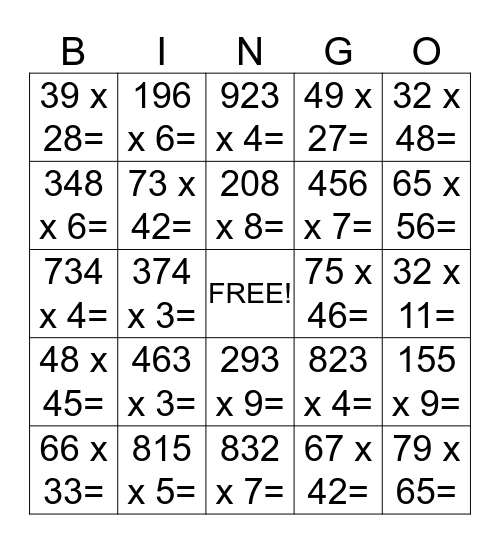# MultiplicationThis bingo card has a free space and 64 words: 102 x 6=, 126 x 6=, 13 x 16=, 155 x 9=, 168 x 3=, 194 x 8=, 196 x 6=, 20 x 10=, 208 x 8=, 25 x 97=, 293 x 9=, 305 x 2=, 32 x 11=, 32 x 48=, 325 x 7=, 348 x 6=, 374 x 3=, 39 x 28=, 432 x 5=, 456 x 7=, 463 x 3=, 48 x 45=, 49 x 27=, 49 x 72=, 494 x 2=, 53 x 27=, 54 x 12=, 54 x 21=, 55 x 72=, 56 x 15=, 604 x 4=, 611 x 2=, 626 x 3=, 630 x 5=, 65 x 56=, 66 x 33=, 67 x 14=, 67 x 42=, 721 x 9=, 73 x 42=, 734 x 4=, 75 x 46=, 76 x 14=, 76 x 86=, 78 x 17=, 79 x 65=, 815 x 5=, 817 x 6=, 823 x 4=, 83 x 24=, 83 x 33=, 83 x 81=, 832 x 7=, 84 x 62=, 841 x 5=, 886 x 2=, 923 x 4=, 94 x 49=, 96 x 51=, 96 x 52=, 980 x 7=, 987 x 6=, 99 x 41= and 999 x 3=.

## Play Online